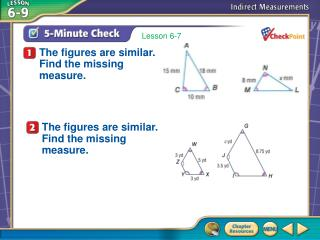# 5-Minute Check 1 - PowerPoint PPT PresentationDownload Presentation5-Minute Check 1

5-Minute Check 1Download Presentation## 5-Minute Check 1

- - - - - - - - - - - - - - - - - - - - - - - - - - - E N D - - - - - - - - - - - - - - - - - - - - - - - - - - -
##### Presentation Transcript

1. A B C D Lesson 6-7 The figures are similar. Find the missing measure. The figures are similar. Find the missing measure. 5-Minute Check 1

2. Splash Screen

3. You have already used similar figures to determine missing measures. (Lesson 6–7) • Solve problems involving indirect measurement using shadow reckoning. • Solve problems using surveying methods. Then/Now

4. indirect measurement Using the properties of similar triangles to find measurements that are difficult to measure directly Vocabulary

5. Use Shadow Reckoning MONUMENTSSuppose the San Jacinto Monument in LaPorte, Texas, casts a shadow of 285 feet. At the same time a nearby tourist, who is 5 feet tall, casts a 2.5-foot shadow. How tall is the San Jacinto Monument? UnderstandYou know the lengths of the shadows and the height of the tourist. You need to find the height of the San Jacinto Monument. Plan Write and solve a proportion. Example 1

6. Use Shadow Reckoning (Drawings below not to scale). How tall is this? 5 feet 285 feet 2.5 feet Example 1

7. tourist’s height tourist’s shadow monument’s height monument’s shadow Use Shadow Reckoning Solve 2.5 ● h = 285 ● 5 Find the cross products. 2.5h = 1425 Multiply. h = 570 Divide each side by 2.5. Answer: The height of the San Jacinto Monument is 570 feet. Example 1

8. Use Shadow Reckoning CheckThe tourist’s height is 2 times the length of his or her shadow. The monument should be 2 times its shadow, or 2 ●285, which is 570 feet. Example 1

9. A B C D BUILDINGA man standing near a building casts a 2.5-foot shadow at the same time the building casts a 200-foot shadow. If the man is 6 feet tall, how tall is the building? A. 1200 feet B. 480 feet C. 83.3 feet D. 13.3 feet Example 1

10. Find Missing Measures MAPS In the figure, ΔPQT ~ ΔPRS. Find the distance across the lake. Since the figures are similar, corresponding sides are proportional. Example 2

11. Find Missing Measures Write a proportion. PR = 60, PQ = 25, RS = x, and QT = 12. Find the cross products. Multiply. Divide each side by 25. Answer: The distance across the lake is 28.8 meters. Example 2

12. A B C D MAPS In the figure, ΔMNO is similar to ΔQPO. Find the distance across the park. A. 1.9 mi B. 3.1 mi C. 4.8 mi D. 5.0 mi Example 2

13. End of the Lesson